# Lesson 4 Variables in JavaScript

In this Lesson, we are going to focus on JavaScript variables and their syntax.
We are also going to learn how you can recall variable and how you can change its value.

Variables are a concept that exists in every programming language and the basic concept is always the same.
A variable is a container that has a name on it and inside of that container we store some bit of data.

We saw the basic primitive types and things like strings, Boolean, and numbers in the previous lesson.
Variables are just a place, a container, or a jar where we can put one of those primitives in it and give it a name that we refer later. So variables are really important they let us store some data and recall it later.

Let suppose we have a website with user login functionality if some user logged in to that site then our site would know the name of that user and assign variable to the user who just logged in to our site, and then as soon as someone logs out, its then set to be empty or false or something.

Another example we have another variable for our Ludo game called ‘current turn’ and it would switch between player 1 and player 2 and that variable is in charge of knowing or keeping track of which player is supposed to roll dice.

So the syntax for variables in JS is very simple.

Like this: `var your variable name = value;`

## Example:

So here is some example where we have initialized 3 variables with the name num1, num2 and total and assign some number to num1 and num2 and add the sum in total variable.

## Code

``````var num1 = 7;
var num2 = 6;
var total = number1 + number2;
"the total is:" +total;
``````

## Output

You can see here we used two variables like num1 and num2 to hold values, and then created another variable ‘total’ and added values of variable num1 and num2 in total variable like this (total = num1 + num2).
And we have got the total which is 13.
The concept of programming is much similar to algebra.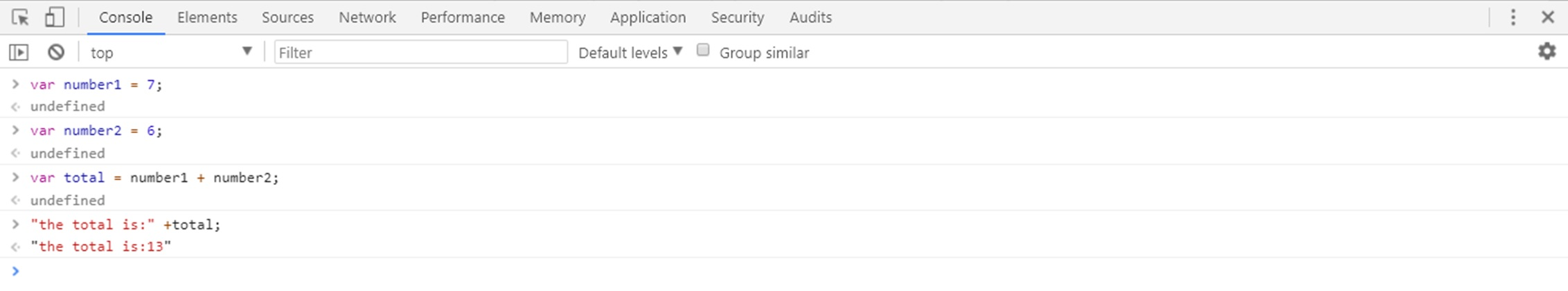Here is another experiment with these var in the Google Chrome Developer console to make it understand more.
So go and declare some variable in chrome console.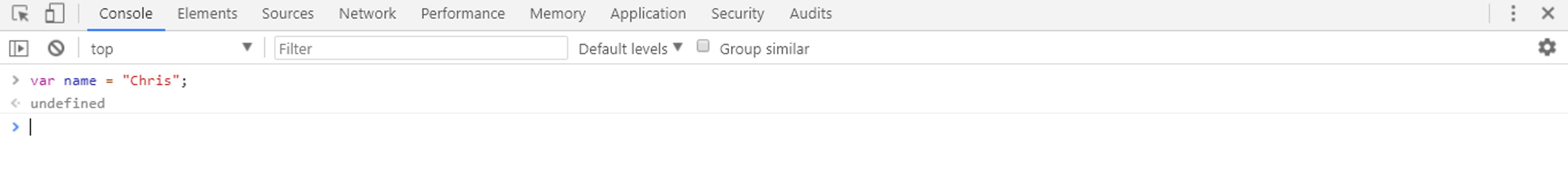You can see in the screenshot we have declared ‘name’ variable and set its value to ‘Chris’ and It says undefined as soon as we hit the enter.
But whenever I will write the name, it will give the name ‘Chris’.
Because it has stored the variable.

JavaScript gives us the authority to change the value of the name variable, to do that reassign the name variable, here is an example below.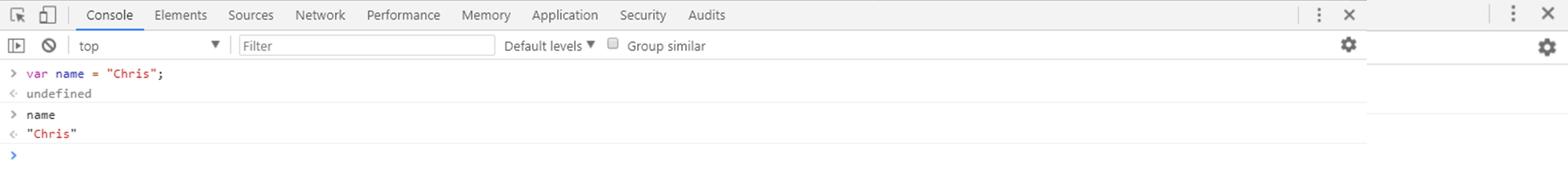Now if I ask for the name, it will say Martin: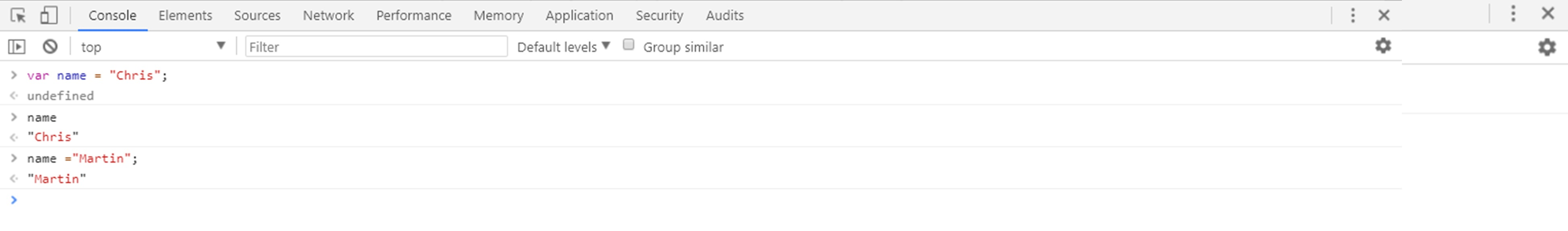So that it is the important part of a variable that it can change the variable value.
Here is another example where we recalling the value of name and then concatenating it with some string.
Here I am creating one more variable name pet and inside the pet container, we stored the value catty.
Now if I write “Hey my friend ”+ pet, it will give: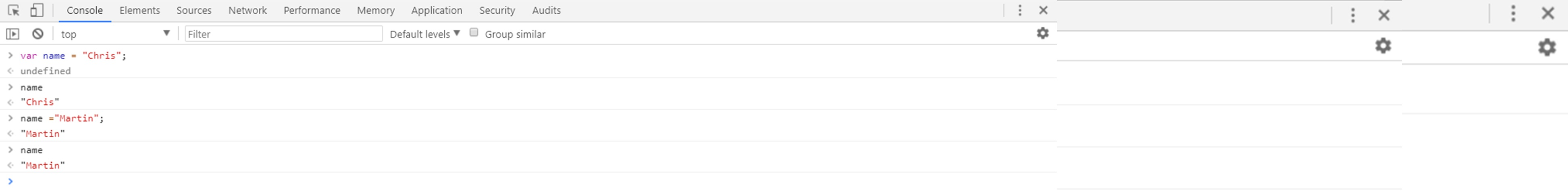So variables are used to store data values.
Now let me show you how numbers are added to the container and how they execute.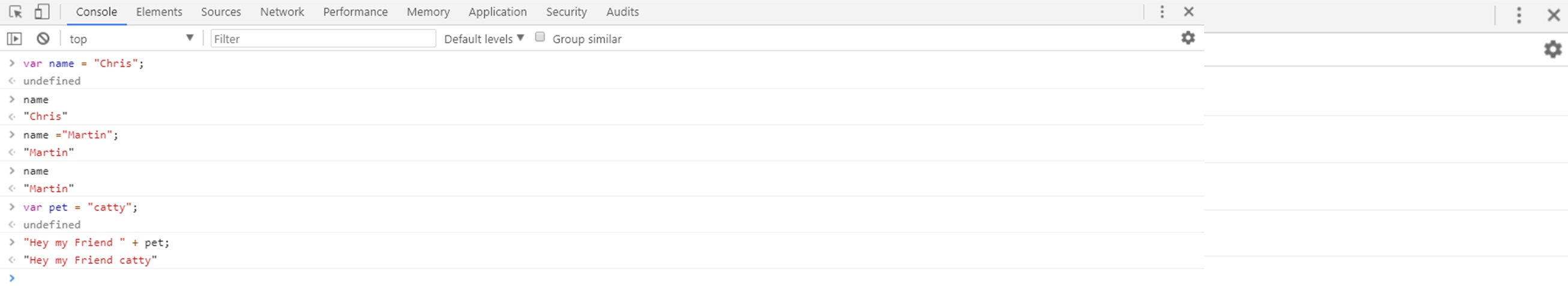And if I write ‘num’, variable then I will get 25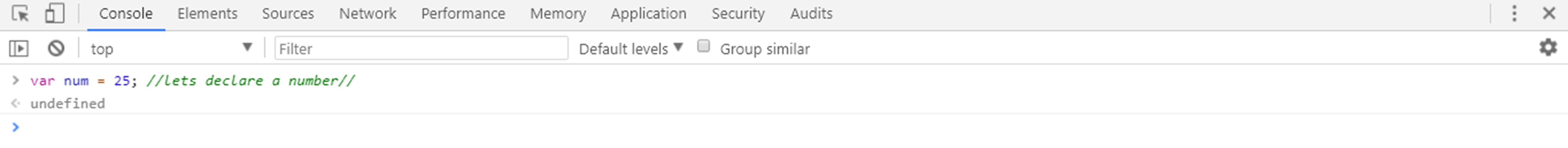With this, we can do the math as well. Let’s see how we can do: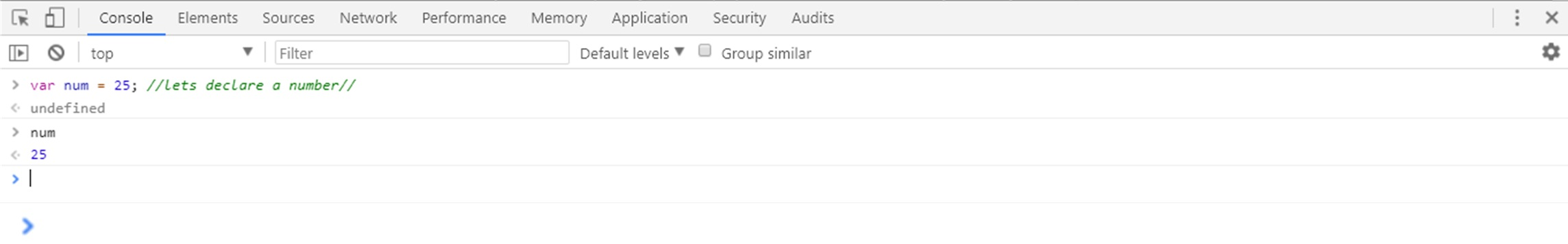So another important concept about the variable in JavaScript is that we can change the value and we can also change the type of the variable.
See this: Let’s suppose that I want to change the value of num to string “thirty”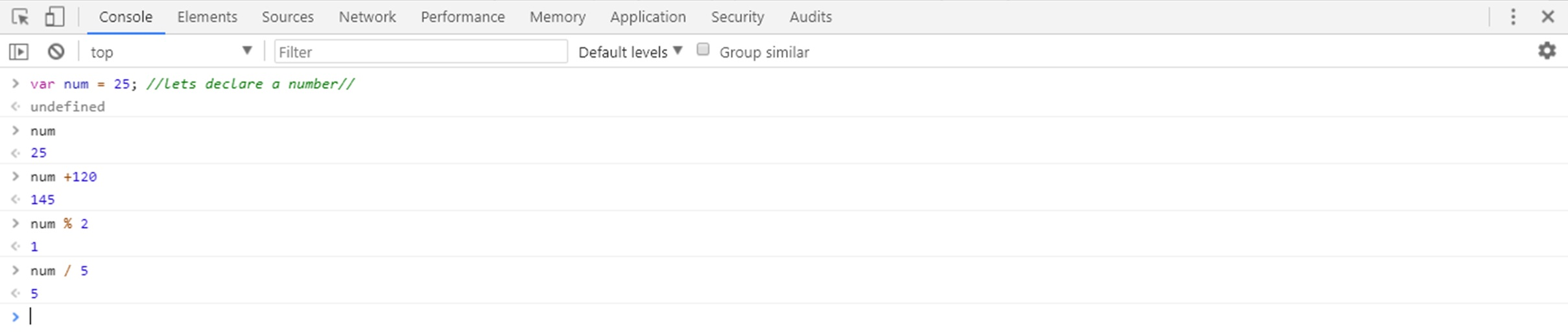JavaScript gives us the dynamic type that means you can change number into alphabets or other characters.
In other languages that is a problem and you can’t do that.
In this Lesson, we have focused on JavaScript variables and their syntax.
We have also learned how you can recall variable and how you can change its value.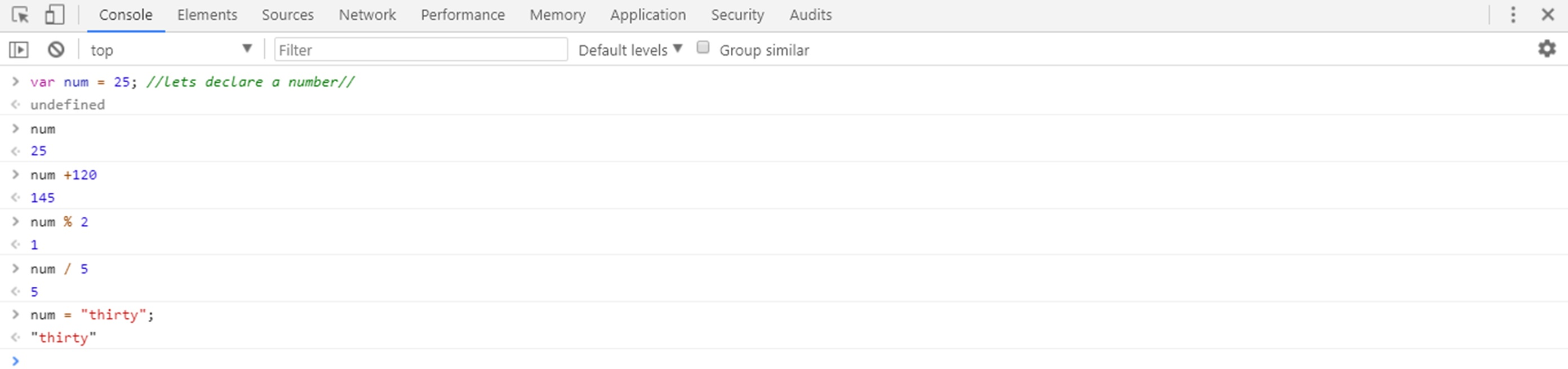## AUTHORGood tutorial! But if you're learning about variables, I would let people know to NOT use `var`. Instead, use `let` and `const`.over 1 year ago